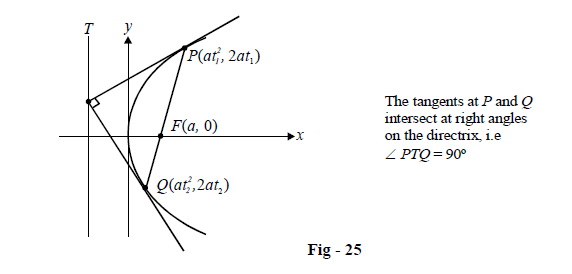# Tangents to Parabolas

Go back to  'Parabola'

In this section, we discuss tangents to parabolas and various properties that they will satisfy. As always, $${y^2} = 4ax$$ is the parabola used for illustration but the discussion can obviously be generalised.

TANGENT AT $$P\left( {{x_1}{y_1}} \right)$$ : Suppose a point $$P\left( {{x_1}{y_1}} \right)$$ is given on the parabola, and we need to find the equation of the tangent at P. The slope of the tangent can easily be evaluated by differentiating the equation of the parabola :

\begin{align}&\qquad \quad \;\;{y^2} = 4ax\\\\&\Rightarrow \qquad 2y\frac{{dy}}{{dx}} = 4a\\\\&\Rightarrow \qquad \frac{{dy}}{{dx}} = \frac{{2a}}{y}\\\\&\Rightarrow \qquad {\left. {\frac{{dy}}{{dx}}} \right|_{{\rm{at }}P({x_1},{y_1})}} = \frac{{2a}}{{{y_1}}}\end{align}

Thus, the equation of the tangent is

\begin{align}&\qquad \;\;y - {y_1} = \frac{{2a}}{{{y_1}}}(x - {x_1})\\\\&\Rightarrow \quad y{y_1} = 2ax - 2a{x_1} + y_1^2\\\\& \Rightarrow \quad y{y_1} = 2ax - 2a{x_1} + 4ax_1^2 \qquad (\because \; y_1^2 = 4a{x_1})\\\\&\Rightarrow \quad \boxed{{y{y_1} = 2a(x + {x_1})}}\qquad \qquad \qquad \dots(1)\end{align}

This equation is sometimes written concisely as $$T({x_1},{y_1}) = 0$$ where the interpretation of T is understood to be as in (1)

TANGENT AT  $${\bf{P}}({\bf{a}}{{\bf{t}}^2},{\rm{ }}{\bf{2at}})$$:  A lot many times, the point P is specified in its parametric form and we need to find the equation of the tangent in terms of the parameter t. This can be done by replacing $$\left( {{x_1},{\rm{ }}{y_1}} \right)$$ with $$\left( {a{t^2},{\rm{ }}2at} \right)$$ in the equation of the tangent just obtained :

\begin{align}&\qquad \;\;y{y_1} = 2a(x + {x_1})\\\\&\Rightarrow \quad 2aty = 2a(x + a{t^2})\\\\&\Rightarrow \quad \boxed{{ty = x + a{t^2}}}\end{align}

This form of the tangent is very widely used and it is best for you to commit it to memory.

TANGENT OF SLOPE m Suppose that we are required to find the equation of the tangent with slope m.We did so in the last section when we found the intersection of the line $$y = mx + c$$ with the parabola and obtained the condition for tangency to be

$\fbox{$${c = \frac{a}{m}}$$}$

The equation of the tangent thus obtained was

$\fbox{$${y = mx + \frac{a}{m}}$$}$

This line is a tangent to the parabola for all non-zero values of m.

The same equation can be obtained using a derivatives approach also. You are urged to do so as an exercise.

Example – 16

Show that the tangents at the extremities of any focal chord of a parabola intersect at right angles at the directrix.

Solution: Let the extremities of the focal chord be $${t_1}\,and\,{\rm{ }}{t_2}$$ so that $${t_1}{t_2} = {\rm{ }}-1$$. The equations to the tangents at $${t_1}\,and\,{\rm{ }}{t_2}$$ are

\begin{align}&{t_1}y = x + at_1^2\\&{t_2}y = x + at_2^2\end{align}

The intersection point can easily be evaluated by solving these two equations to be $$(a{t_1}{t_2},a({t_1} + {t_2})) = ( - a,a({t_1} + {t_2}))$$ since $${t_1}{t_2} = - 1.$$ Since the x-coordinate of the point of intersection is –a, it lies on the directrix $$x + a = 0.$$ Also, note that the slopes of the two tangents are \begin{align}{{m}_{1}}=\frac{1}{{{t}_{1}}}\,\rm{ }and\,\text{ }{{m}_{2}}=\frac{1}{{{t}_{2}}}\text{ }so\text{ }that~\text{ }{{m}_{1}}{{m}_{2}}=\frac{1}{{{t}_{1}}{{t}_{2}}}=-1.\end{align} Thus, the tangents intersect at right angles on the directrix.Note that T will also be the point at which the circle drawn on PQ as diameter touches the directrix, since $$\angle PTQ$$ is 90° which then becomes an angle in the semi circle.

POINT OF INTERSECTION OF TANGENTS AT t1 and t2: There’s a particular relation we obtained while solving the last example which is important enough to be highlighted explicitly. If tangents are drawn to $${y^2} = 4ax\;$$ at $${t_1}{\text{ and}}\;{t_2}$$, their point of intersection will be

$\fbox{$${a{t_1}{t_2},\,a({t_1} + {t_2})}$$}$